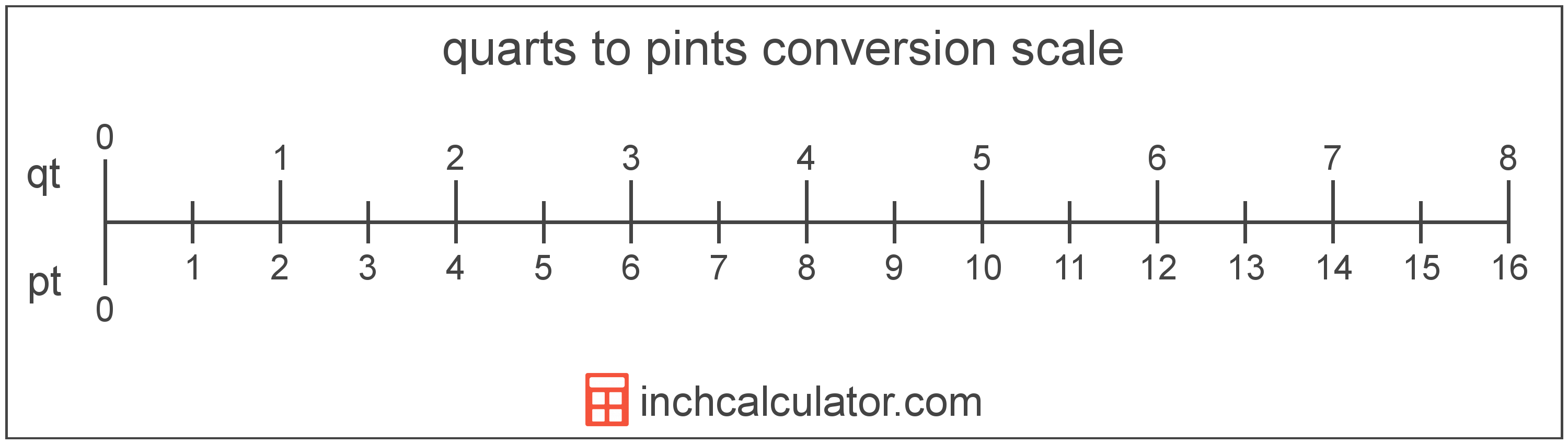# Pints to Quarts Conversion

Enter the volume in pints below to get the value converted to quarts.

Results in Quarts:1 pt = 0.5 qt
Do you want to convert quarts to pints?

## How to Convert Pints to QuartsTo convert a pint measurement to a quart measurement, divide the volume by the conversion ratio.

Since one quart is equal to 2 pints, you can use this simple formula to convert:

quarts = pints ÷ 2

The volume in quarts is equal to the pints divided by 2.

For example, here's how to convert 5 pints to quarts using the formula above.
5 pt = (5 ÷ 2) = 2.5 qt

## Pints

The US liquid pint is a unit of fluid volume equal to one-eighth of a gallon, one-half of a quart, or two cups. The liquid pint should not be confused with the dry pint (US) or the imperial pint, which are different units.

The pint is a US customary unit of volume. Pints can be abbreviated as pt; for example, 1 pint can be written as 1 pt.

## Quarts

The US liquid quart is a unit of fluid volume equal to one fourth of a gallon, two pints, or four cups. The liquid quart should not be confused with the dry quart (US) or the imperial quart, which are different units.

The quart is a US customary unit of volume. Quarts can be abbreviated as qt; for example, 1 quart can be written as 1 qt.

## Pint to Quart Conversion Table

Pint measurements converted to quarts
Pints Quarts
1 pt 0.5 qt
2 pt 1 qt
3 pt 1.5 qt
4 pt 2 qt
5 pt 2.5 qt
6 pt 3 qt
7 pt 3.5 qt
8 pt 4 qt
9 pt 4.5 qt
10 pt 5 qt
11 pt 5.5 qt
12 pt 6 qt
13 pt 6.5 qt
14 pt 7 qt
15 pt 7.5 qt
16 pt 8 qt
17 pt 8.5 qt
18 pt 9 qt
19 pt 9.5 qt
20 pt 10 qt
21 pt 10.5 qt
22 pt 11 qt
23 pt 11.5 qt
24 pt 12 qt
25 pt 12.5 qt
26 pt 13 qt
27 pt 13.5 qt
28 pt 14 qt
29 pt 14.5 qt
30 pt 15 qt
31 pt 15.5 qt
32 pt 16 qt
33 pt 16.5 qt
34 pt 17 qt
35 pt 17.5 qt
36 pt 18 qt
37 pt 18.5 qt
38 pt 19 qt
39 pt 19.5 qt
40 pt 20 qt